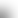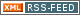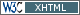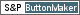www.LinuxHowtos.org howtos, tips&tricks and tutorials for linuxfrom small one page howto to huge articles all in one place

search text in:

Poll
Which filesystem do you use?

poll results

using iotop to find disk usage hogs

using iotop to find disk usage hogs

words:

887

views:

169845

userrating:

average rating: 1.7 (102 votes) (1=very good 6=terrible)

May 25th. 2007:
Words

486

Views

249272

Workaround and fixes for the current Core Dump Handling vulnerability affected kernels

Workaround and fixes for the current Core Dump Handling vulnerability affected kernels

words:

161

views:

135591

userrating:

average rating: 1.4 (41 votes) (1=very good 6=terrible)

April, 26th. 2006:

You are here: manpages

# complex.h

Section: POSIX Programmer's Manual (0P)
Updated: 2013

## PROLOG

This manual page is part of the POSIX Programmer's Manual. The Linux implementation of this interface may differ (consult the corresponding Linux manual page for details of Linux behavior), or the interface may not be implemented on Linux.

## NAME

complex.h --- complex arithmetic

## SYNOPSIS

```#include <complex.h>
```

## DESCRIPTION

The functionality described on this reference page is aligned with the ISO C standard. Any conflict between the requirements described here and the ISO C standard is unintentional. This volume of POSIX.1-2008 defers to the ISO C standard. The <complex.h> header shall define the following macros:
complex
Expands to _Complex.
_Complex_I
Expands to a constant expression of type const float _Complex, with the value of the imaginary unit (that is, a number i such that i2=-1).
imaginary
Expands to _Imaginary.
_Imaginary_I
Expands to a constant expression of type const float _Imaginary with the value of the imaginary unit.
I
Expands to either _Imaginary_I or _Complex_I. If _Imaginary_I is not defined, I expands to _Complex_I. The macros imaginary and _Imaginary_I shall be defined if and only if the implementation supports imaginary types. An application may undefine and then, perhaps, redefine the complex, imaginary, and I macros. The following shall be declared as functions and may also be defined as macros. Function prototypes shall be provided.

```
double               cabs(double complex);
float                cabsf(float complex);
long double          cabsl(long double complex);
double complex       cacos(double complex);
float complex        cacosf(float complex);
double complex       cacosh(double complex);
float complex        cacoshf(float complex);
long double complex  cacoshl(long double complex);
long double complex  cacosl(long double complex);
double               carg(double complex);
float                cargf(float complex);
long double          cargl(long double complex);
double complex       casin(double complex);
float complex        casinf(float complex);
double complex       casinh(double complex);
float complex        casinhf(float complex);
long double complex  casinhl(long double complex);
long double complex  casinl(long double complex);
double complex       catan(double complex);
float complex        catanf(float complex);
double complex       catanh(double complex);
float complex        catanhf(float complex);
long double complex  catanhl(long double complex);
long double complex  catanl(long double complex);
double complex       ccos(double complex);
float complex        ccosf(float complex);
double complex       ccosh(double complex);
float complex        ccoshf(float complex);
long double complex  ccoshl(long double complex);
long double complex  ccosl(long double complex);
double complex       cexp(double complex);
float complex        cexpf(float complex);
long double complex  cexpl(long double complex);
double               cimag(double complex);
float                cimagf(float complex);
long double          cimagl(long double complex);
double complex       clog(double complex);
float complex        clogf(float complex);
long double complex  clogl(long double complex);
double complex       conj(double complex);
float complex        conjf(float complex);
long double complex  conjl(long double complex);
double complex       cpow(double complex, double complex);
float complex        cpowf(float complex, float complex);
long double complex  cpowl(long double complex, long double complex);
double complex       cproj(double complex);
float complex        cprojf(float complex);
long double complex  cprojl(long double complex);
double               creal(double complex);
float                crealf(float complex);
long double          creall(long double complex);
double complex       csin(double complex);
float complex        csinf(float complex);
double complex       csinh(double complex);
float complex        csinhf(float complex);
long double complex  csinhl(long double complex);
long double complex  csinl(long double complex);
double complex       csqrt(double complex);
float complex        csqrtf(float complex);
long double complex  csqrtl(long double complex);
double complex       ctan(double complex);
float complex        ctanf(float complex);
double complex       ctanh(double complex);
float complex        ctanhf(float complex);
long double complex  ctanhl(long double complex);
long double complex  ctanl(long double complex);
```

The following sections are informative.

## APPLICATION USAGE

Values are interpreted as radians, not degrees.

## RATIONALE

The choice of I instead of i for the imaginary unit concedes to the widespread use of the identifier i for other purposes. The application can use a different identifier, say j, for the imaginary unit by following the inclusion of the <complex.h> header with:

```
#undef I
#define j _Imaginary_I
```
An I suffix to designate imaginary constants is not required, as multiplication by I provides a sufficiently convenient and more generally useful notation for imaginary terms. The corresponding real type for the imaginary unit is float, so that use of I for algorithmic or notational convenience will not result in widening types. On systems with imaginary types, the application has the ability to control whether use of the macro I introduces an imaginary type, by explicitly defining I to be _Imaginary_I or _Complex_I. Disallowing imaginary types is useful for some applications intended to run on implementations without support for such types. The macro _Imaginary_I provides a test for whether imaginary types are supported. The cis() function (cos(x) + I*sin(x)) was considered but rejected because its implementation is easy and straightforward, even though some implementations could compute sine and cosine more efficiently in tandem.

## FUTURE DIRECTIONS

The following function names and the same names suffixed with f or l are reserved for future use, and may be added to the declarations in the <complex.h> header.

 ```cerf() cerfc() cexp2() ``` cexpm1() clog10() clog1p() clog2() clgamma() ctgamma()

The System Interfaces volume of POSIX.1-2008, cabs(), cacos(), cacosh(), carg(), casin(), casinh(), catan(), catanh(), ccos(), ccosh(), cexp(), cimag(), clog(), conj(), cpow(), cproj(), creal(), csin(), csinh(), csqrt(), ctan(), ctanh()

Portions of this text are reprinted and reproduced in electronic form from IEEE Std 1003.1, 2013 Edition, Standard for Information Technology -- Portable Operating System Interface (POSIX), The Open Group Base Specifications Issue 7, Copyright (C) 2013 by the Institute of Electrical and Electronics Engineers, Inc and The Open Group. (This is POSIX.1-2008 with the 2013 Technical Corrigendum 1 applied.) In the event of any discrepancy between this version and the original IEEE and The Open Group Standard, the original IEEE and The Open Group Standard is the referee document. The original Standard can be obtained online at http://www.unix.org/online.html .

Any typographical or formatting errors that appear in this page are most likely to have been introduced during the conversion of the source files to man page format. To report such errors, see https://www.kernel.org/doc/man-pages/reporting_bugs.html .

## Index

PROLOG
NAME
SYNOPSIS
DESCRIPTION
APPLICATION USAGE
RATIONALE
FUTURE DIRECTIONS||- Powered by- Running on-
Copyright 2004-2020 Sascha Nitsch Unternehmensberatung GmbH::- Level Triple-A Conformance to Web Content Accessibility Guidelines 1.0 -
- Copyright and legal notices -